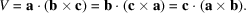International
Tables for
Crystallography
Volume A
Space-group symmetry
Edited by M. I. Aroyo

International Tables for Crystallography (2016). Vol. A, ch. 1.3, p. 24

## Section 1.3.2.3. Unit cells

B. Souvigniera*

aRadboud University Nijmegen, Faculty of Science, Mathematics and Computing Science, Institute for Mathematics, Astrophysics and Particle Physics, Postbus 9010, 6500 GL Nijmegen, The Netherlands
Correspondence e-mail: souvi@math.ru.nl

#### 1.3.2.3. Unit cells

| top | pdf |

A lattice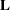can be used to subdivideinto cells of finite volume which all have the same shape. The idea is to define a suitable subset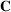ofsuch that the translates ofby the vectors incoverwithout overlapping. Such a subsetis called a unit cell of L, or, in the more mathematically inclined literature, a fundamental domain ofwith respect to. Two standard constructions for such unit cells are the primitive unit cell and the Voronoï domain (which is also known by many other names).

#### Definition

Letbe a lattice inwith lattice basis.

 (i) The set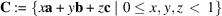is called the primitive unit cell ofwith respect to the basis. The primitive unit cell is the parallelepiped spanned by the vectors of the given basis. (ii) The set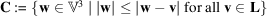is called the Voronoï domain or Dirichlet domain or Wigner–Seitz cell or Wirkungsbereich or first Brillouin zone (for the case of reciprocal lattices in dual space, see Section 1.3.2.5) of(around the origin). The Voronoï domain consists of those points ofthat are closer to the origin than to any other lattice point of.

See Fig. 1.3.2.2for examples of these two types of unit cells in two-dimensional space.Figure 1.3.2.2 | top | pdf |Voronoï domains and primitive unit cells for a rectangular lattice (a) and an oblique lattice (b).

It should be noted that the attribute primitive' for a unit cell is often omitted. The term unit cell' then either denotes a primitive unit cell in the sense of the definition above or a slight generalization of this, namely a cell spanned by vectors a, b, c which are not necessarily a lattice basis. This will be discussed in detail in the next section. If a unit cell in the even more general sense of a cell whose translates cover the whole space without overlap (thus including e.g. Voronoï domains) is meant, this should be indicated by the context.

The construction of the Voronoï domain is independent of the basis of, as the Voronoï domain is bounded by planes bisecting the line segment between the origin and a lattice point and perpendicular to this segment. In two-dimensional space, the Voronoï domain is simply bounded by lines, in three-dimensional space it is bounded by planes and more generally it is bounded by (n − 1)-dimensional hyperplanes in n-dimensional space.

The boundaries of the Voronoï domain and its translates overlap, thus in order to get a proper fundamental domain, part of the boundary has to be excluded from the Voronoï domain.

The volume V of the unit cell can be expressed both via the metric tensor and via the cell parameters. One has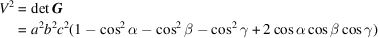and thus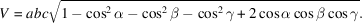Although the cell parameters depend on the chosen lattice basis, the volume of the unit cell is not affected by a transition to a different lattice basis. As remarked in Section 1.3.2.1, two lattice bases are related by an integral basis transformationof determinant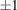and therefore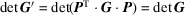, i.e. the determinant of the metric tensor is the same for all lattice bases.

Assuming that the vectorsform a right-handed system, the volume can also be obtained via